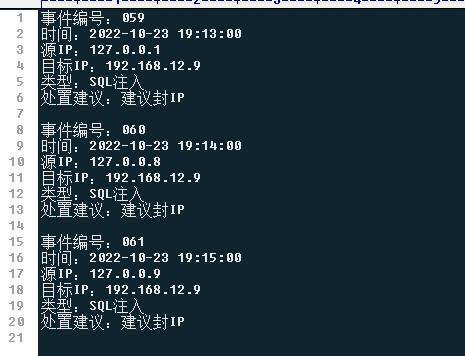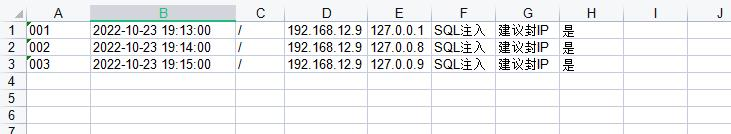﻿ 利用Python将txt自动化写入excel脚本 — BlueCode的自留地

# 利用Python将txt自动化写入excel脚本

txt数据如下：python代码如下：

```import xlwt
import time

# 将读取的数据写入到字典
result = []
dictt = {}
f = open(file, "r", encoding="utf-8")
a = 0
for line in lines: #以下写入字典情况，需要根据具体情况进行调整
a += 1
if a % 7 == 2:
dictt["time"] = line.split("：")[:-1]
elif a % 7 == 3:
dictt["src_ip"] = line.split("：")[:-1]
elif a % 7 == 4:
dictt["dst_ip"] = line.split("：")[:-1]
elif a % 7 == 5:
dictt["attack_type"] = line.split("：")[:-1]
elif a % 7 == 6:
dictt["chuzhi"] = line.split("：")[:-1]
result.append(dictt)
dictt = {}  # 因为每次添加的都是同一个内存到list中去了.dictt每次写入的时候改变了内存中的value,但是地址不变.即是,创建了一次内存空间,只会不断的改变value了,添加到list中的时候value已经改了。所以需要在for循环里面去每次循环都创建一个空的dict，以保证之前添加过的不会被改变。
else:
continue
return result

# 将列表写入到excel表
def write_exec(list):
workbook = xlwt.Workbook(encoding="utf-8", style_compression=0)
# Cell_overwirte_ok 是能够覆盖单元表格的意思。
for i in range(len(list)):  #以下写入表格的具体位置需要根据具体情况进行调整
if i+1 < 10:
num = '00' + str(i+1)
elif i+1 < 100:
num = '0' + str(i+1)
else: num = str(i+1)
sheet.write(i, 0, num)
sheet.write(i, 1, list[i]['time'])
sheet.write(i, 2, '/')
sheet.write(i, 3, list[i]['dst_ip'])
sheet.write(i, 4, list[i]['src_ip'])
sheet.write(i, 5, list[i]['attack_type'])
sheet.write(i, 6, list[i]['chuzhi'])
sheet.write(i, 7, '是') #默认填入'是'
workbook.save(r"./01-攻击事件汇总清单_{}.xls".format(time.strftime('%Y%m%d',time.localtime(time.time())))) #导出的excel文件名

filename = time.strftime('%m%d',time.localtime(time.time())) #写入的txt文件名
file = filename + '.txt'
# print(dictt)
write_exec(dictt)
print('写入完成')```

## 关于博主• Linux文章
• PHP文章
• 随机文章